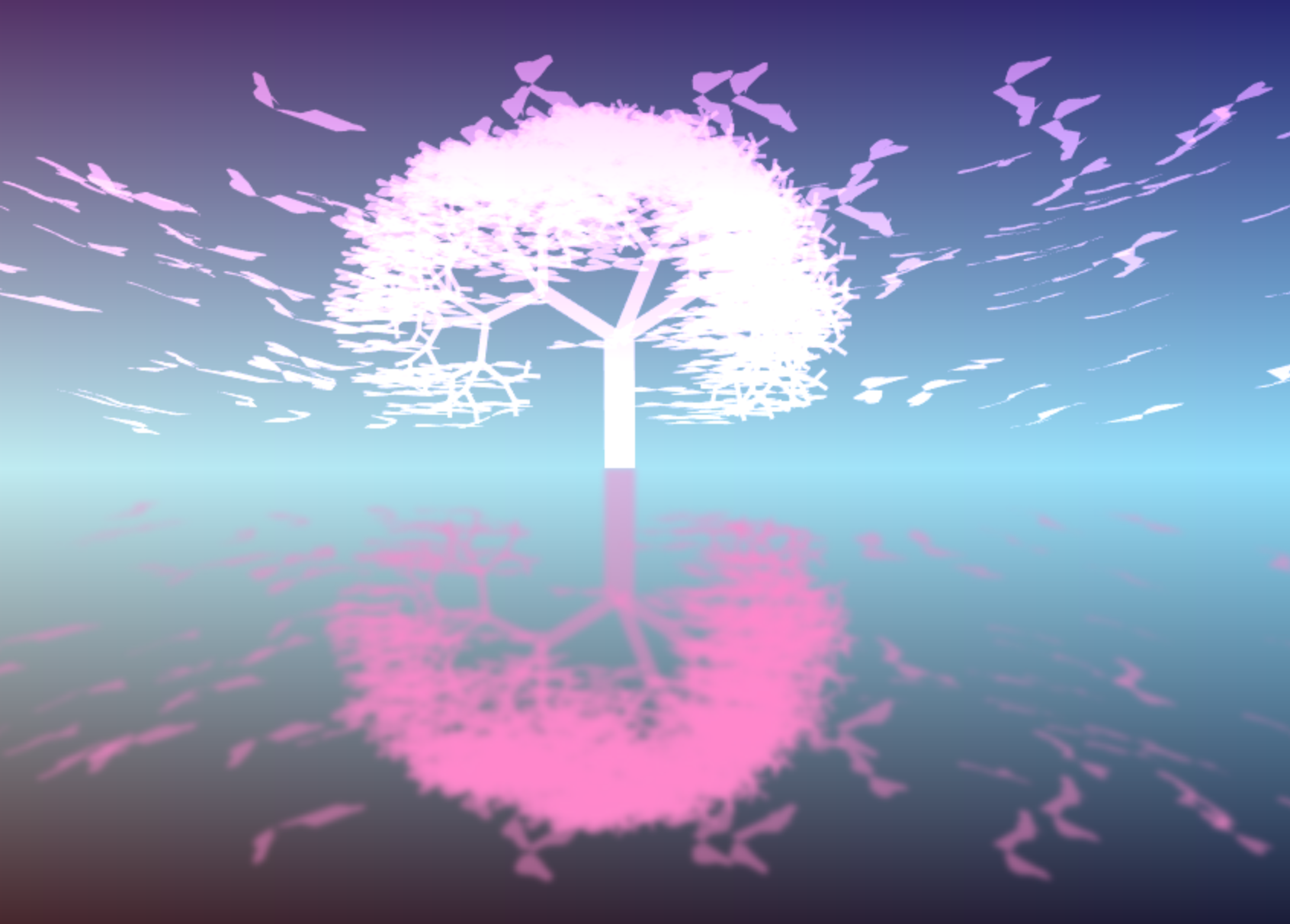# JavaScript Butterfly Sakura 1k postmortem

## blog

As usual, I love to take part on the javascript 1k code golfing competitions and this year was no different, so I managed to do something for 2021 edition the js1024.fun.

The submitted version can be seen directly here: 1k Butterfly Sakura, a slightly fixed and updated version here: 1k Butterfly Sakura - update and the source code: 1k Butterfly Sakura source

I took inspiration on a Unity3D Experiment by Max Gittel I saw in the past, but to recreate it (more or less) in 1k. The original experiment can be seen here in action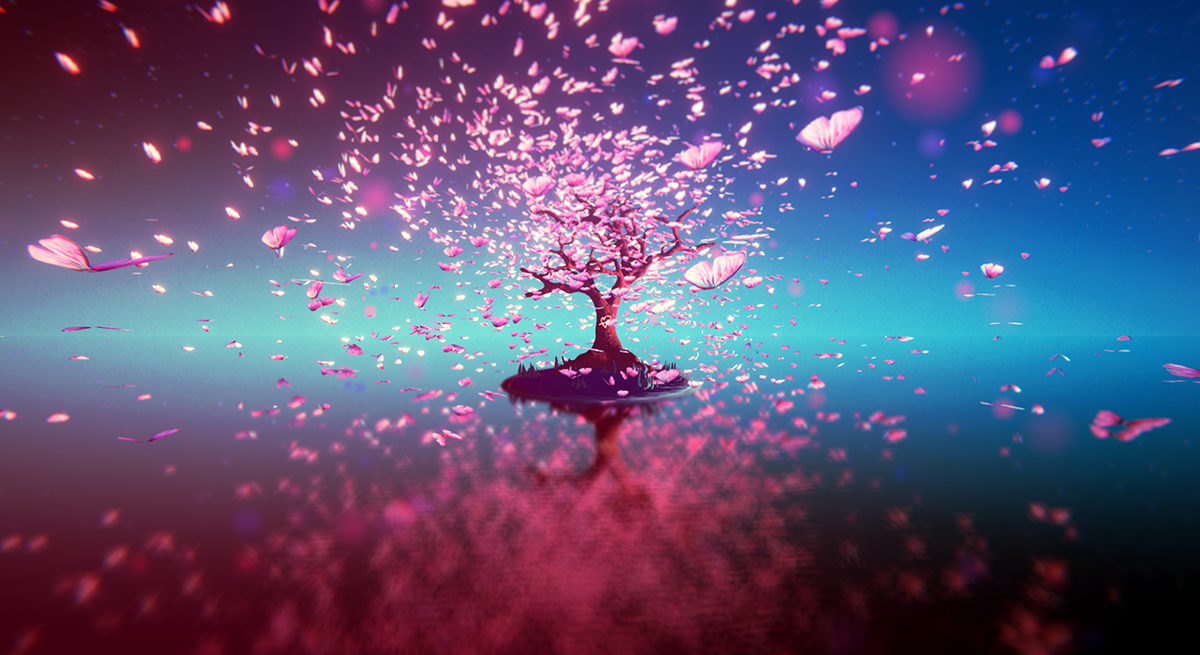I started by trying to recreate the background, first by manually iterating colors - both horitzontally and vertically:

``````for(i = 0; i < N; i++) {
for(j = 0; j < N; j++) {
// vertical factor
f0 = i / (N/2-1)

// horizontal factor
f1 = j / (N-1)

// adding a bit of exponential factor to the vertical color interpolation
f0 = f0*f0*f0

r = (AC * f1 + BC * (1 - f1)) * (1-f0) + CC * f0
g = (AC * f1 + BC * (1 - f1)) * (1-f0) + CC * f0
b = (AC * f1 + BC * (1 - f1)) * (1-f0) + CC * f0
c.fillStyle = 'rgb(' + r + ',' +g+ ',' + b + ')'

c.fillRect(j * a.width/N, i * a.height/N, a.width/N, a.height/N)
}
}
``````

But the results were not very nice and, even if it was not optimized, I was not happy with the amount of bytes it was taking just for the background.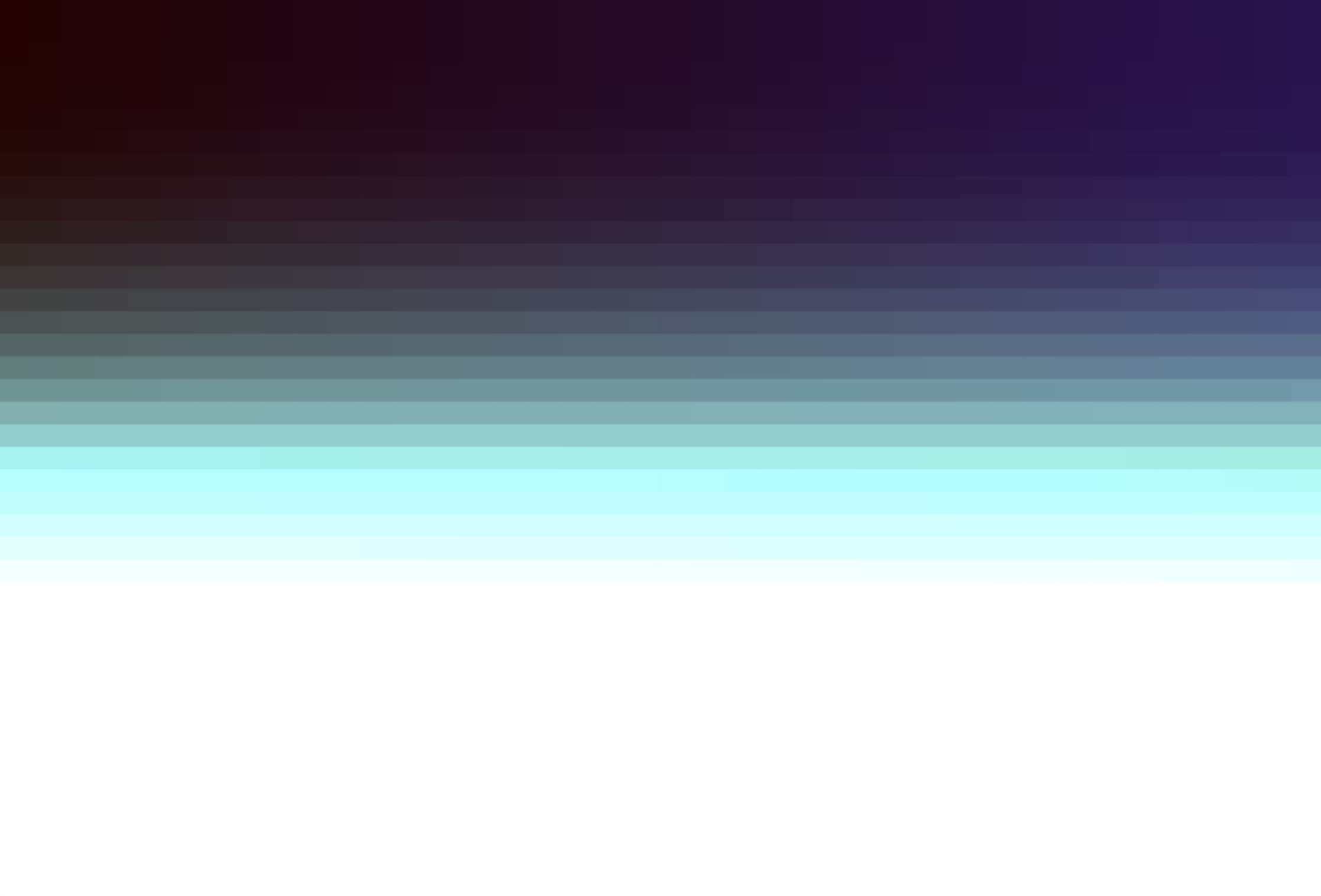So, I decided to change the approach and play with the `createLinearGradient` function of the Canvas `Context2D`. The tricky part was to have a mix of two different gradients, one vertical and one horizontal, but I started by creating the two different gradients:

``````// h = canvas height
E = c.createLinearGradient(0, 0, 0, h)

// w = canvas width
F = c.createLinearGradient(0, 0, w, 0)
``````

Played a bit merging them with alpha (using `globalAlpha`) without too much success but, at the end, using `globalCompositeOperation` and setting it to `lighter` created quite good result and was quite compact in terms of size:

``````c.fillStyle = E
c.fillRect(0, 0, w, h)
c.globalCompositeOperation = 'lighter'
c.fillStyle = F
c.fillRect(0, 0, w, h)
``````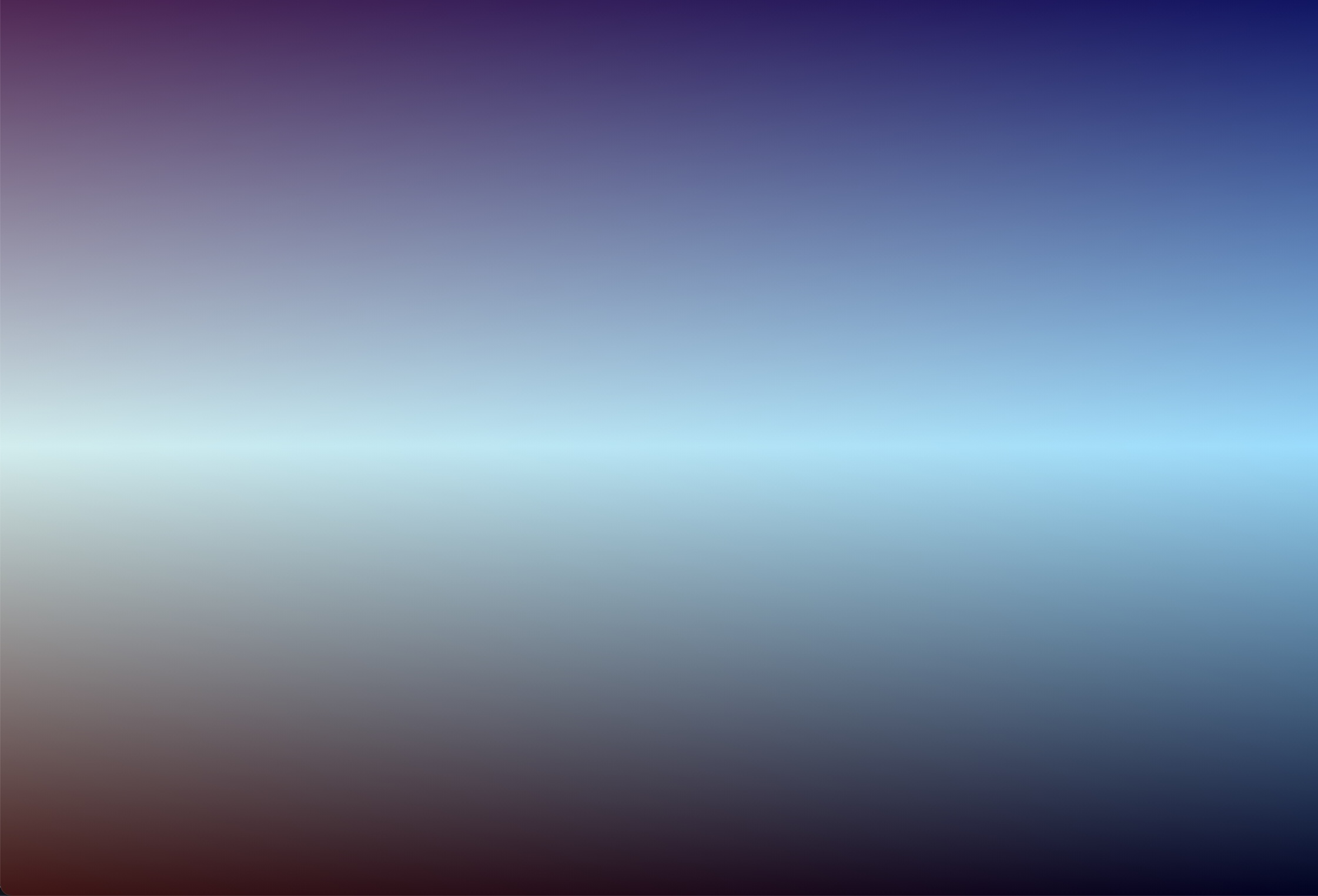Happy with this, I then created a simple main loop with all the elements that had to be drawn:

``````setInterval(_=>{
// clear screen (by setting canvas width it clears the screen for free 😎
a.width = w

// background
...

// draw tree
...

// draw butterflies
...

// blurred reflection
...
})
``````

I started by generating some points of what would be the half shape of the butterfly: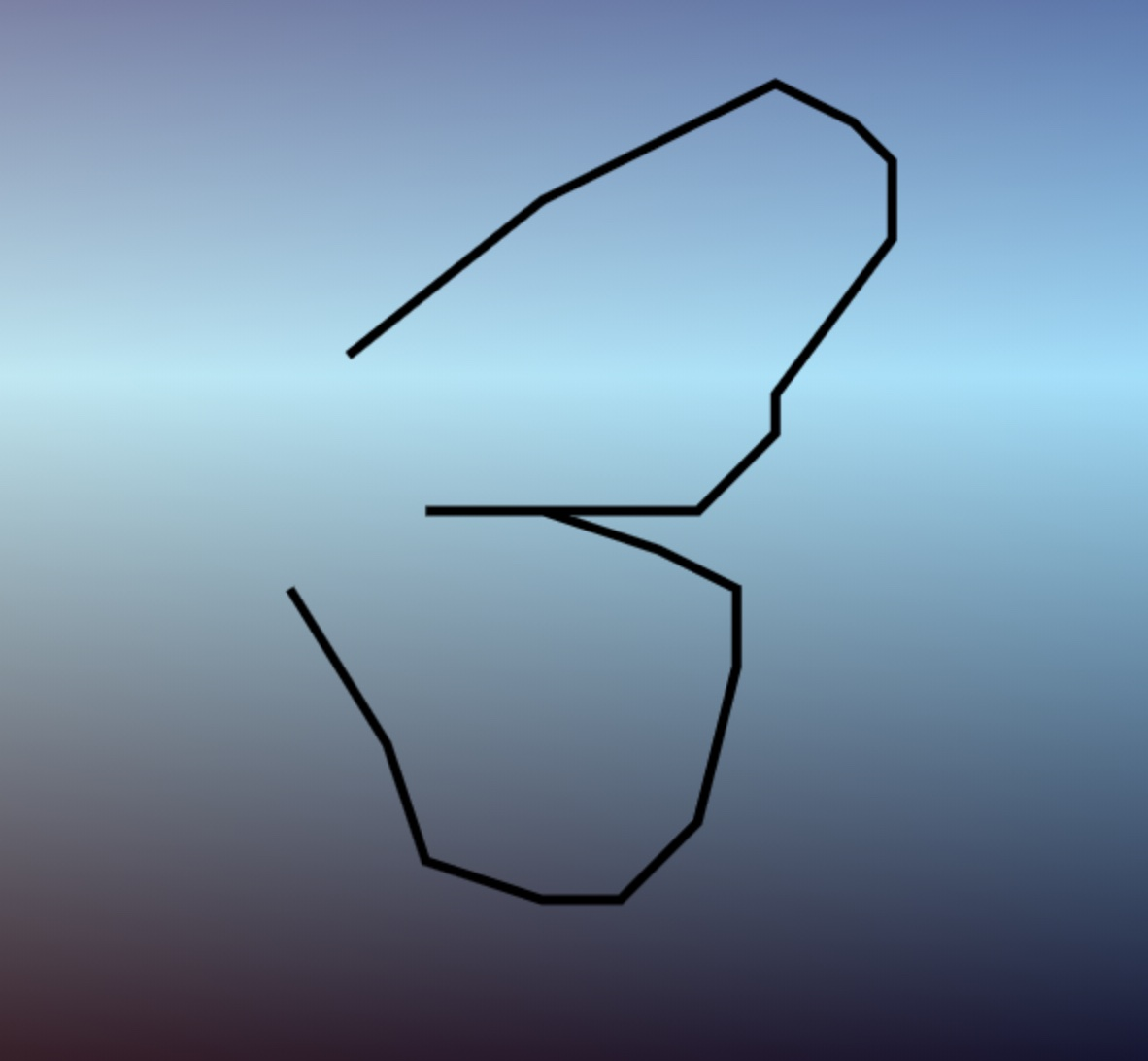``````// butterfly points (x, y, x, y, ...)
points = [30, 19, 20, 10, 13, 6, 8, 5, 4, 6, 3, 8, 3, 12, 8, 20, 8, 23, 10, 25, 13, 26, 27, 26, 20, 27, 15, 28, 11, 31, 10, 35, 12, 42, 16, 47, 21, 46, 26, 44, 29, 38, 30, 31]
``````

Mirroring these points horizontally we can draw the whole shape (not the best drawn butterfly in world though - but hopefully good enough for 1k of javascript code):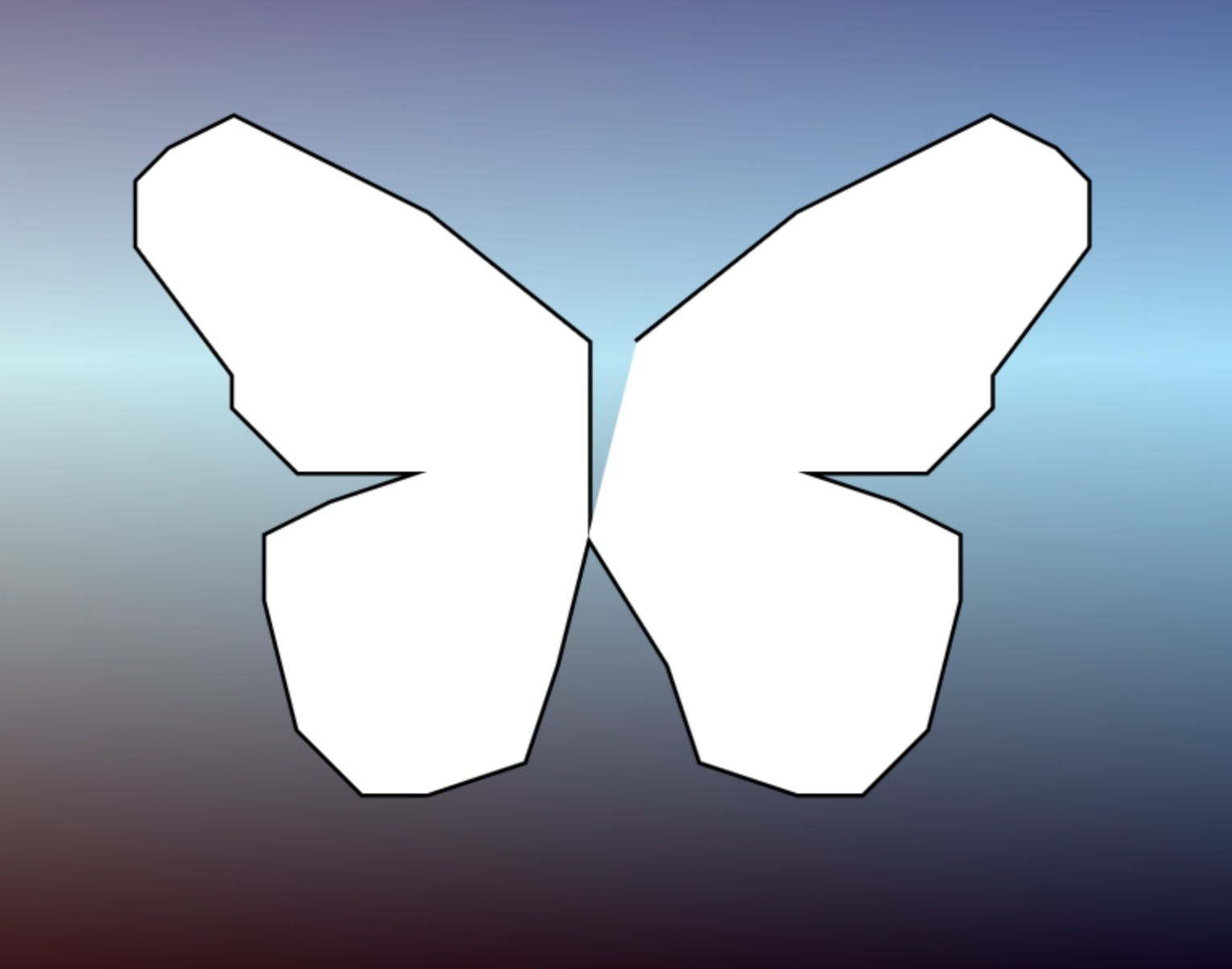For size purposes, points were transformed and encoded as `chars` to be smaller (this is a preprocess, did not end up on the final code):

``````points = [30, 19, 20, 10, 13, 6, 8, 5, 4, 6, 3, 8, 3, 12, 8, 20, 8, 23, 10, 25, 13, 26, 27, 26, 20, 27, 15, 28, 11, 31, 10, 35, 12, 42, 16, 47, 21, 46, 26, 44, 29, 38, 30, 31]

str = ""
for(i = 0; i < points.length; i++) {
// 33 to map it to visible chars after space
// 66 adjust for a lower case ascii string
// points are divided by 2 - losing a bit of precision in the process
// but for space optimization purposes
str += String.fromCharCode(33 + 66 + ((points[i]/2)|0))
}

console.log('p="'+str+'"')
``````

and, when drawing the butterflies, `chars` are decoded again into coordinates by substracting 99 and then multiplying by 20 to get an adequate size.

``````p = "rlmhifgeefdgdigmgnhoipppmpjqhrhtixkzmzpyqvrr"

// iterate twice the amount of data
for(i = 0; i < 88; ) {
// using modulo to loop over the index and avoid overlowing the array
x = -(p.charCodeAt(i++%44) - 99) * 20
y = (p.charCodeAt(i++%44) - 99) * 20

// mirror x coordinate if second half of data
x = i<44 ? x : -630 - x
}
``````

To get some butterflies on the screen, I randomized their position (on the top part only):

``````for(i = 0; i < 607; i++) {
B[i] = [Math.random()*900 - 450, -Math.random()*600]
}
``````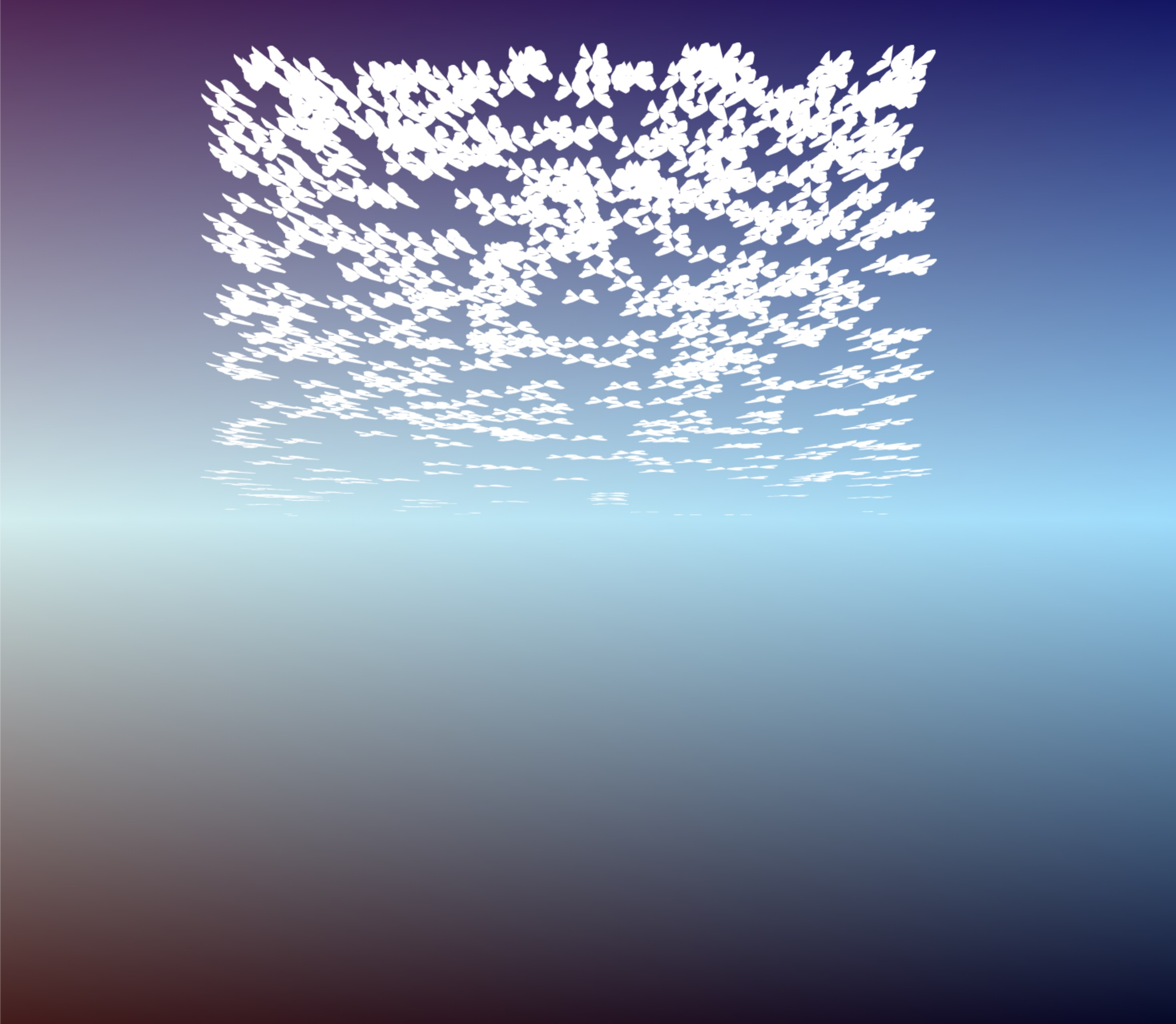For the movement, I wanted them to move in a spiral like path from their starting point: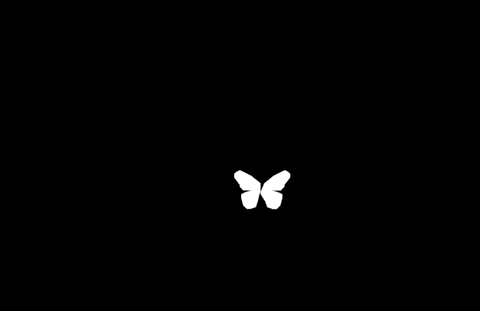but also rotated so they face the direction they are going:

``````  C = Math.cos(f)
S = Math.sin(f)

// rotate the x & z coordinates by the Y axis
xr = x * C - z * S
zr = x * S + z * C
``````

and adding the radius and some `z-offset`, gives us the following result:

``````  C = Math.cos(f)
S = Math.sin(f)

// z-offset 6500 (displace all points further from the camera)
xr = x * C - z * S + 3*radius * C
zr = x * S + z * C + radius * S + 6500
``````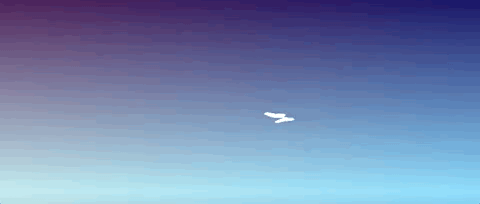Also, we need to add some flapping to the wings, for that instead of having a constant flat `y` coordinate, let’s modify it based on `x` coordinate and time (`t`):

``````  // 80 is the strength of the 'flap'
y = 80 * Math.sin(x / 200 + t * .1)
``````

see the animation in action: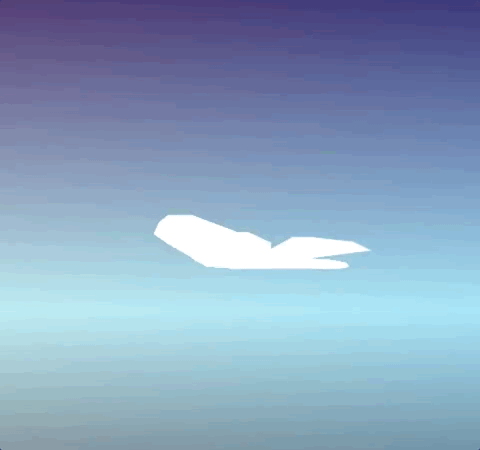and the final animation for each single buttefly: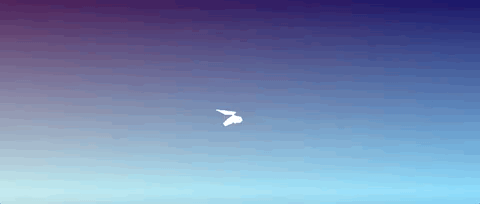If we put all together, with the randomized butterfly positions, this is the result:

``````// draw butterflies
for(k = 0; k < 607; k++) {
c.beginPath()
for(i = 0; i < 80; ) {

x = -(p.charCodeAt(i++%44) - 99) * 20
z = -(p.charCodeAt(i++%44) - 99) * 20

x = i<44 ? x : -630 - x

butterflyTime = Math.max(t - 20 * k, 0)
angle = butterflyTime ? butterflyTime / 80 : 0

y = (butterflyTime ? 80*Math.sin((x + k) / 200 + t * .1) : 0)

radius = Math.min(150 * angle, 2000)
C = Math.cos(angle)
S = Math.sin(angle)

xr = x * C - z * S + 3*radius * C
zr = x * S + z * C + radius * S + 6500

//500 is a FOV for doing the 3D to 2D projection
c.lineTo(
500 * (B[k] + xr) / zr + w/2,
500 * (B[k] + y) / zr + h/2)
}
c.fill()
}
``````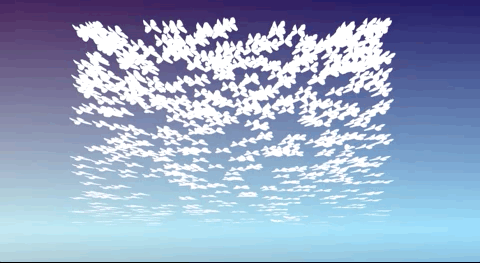Time-based movement of the butterfly is modified with the index and some negative offset, so they do not start moving at the same time:

``````  // index is the butterfly index in the array
butterflyTime = Math.max(t - 20 * index, 0)

//angle is 0 if butterflyTime is below or equal to 0
angle = butterflyTime ? butterflyTime / 80 : 0
``````

Let’s park the butteflies for a moment and let’s focus on the tree. I wanted to do something very simple but with good results. I thought on a recursive tree I used on a presentation I did back on Oracle Code One 2019:

Rendering Art on the Web - A performance compendium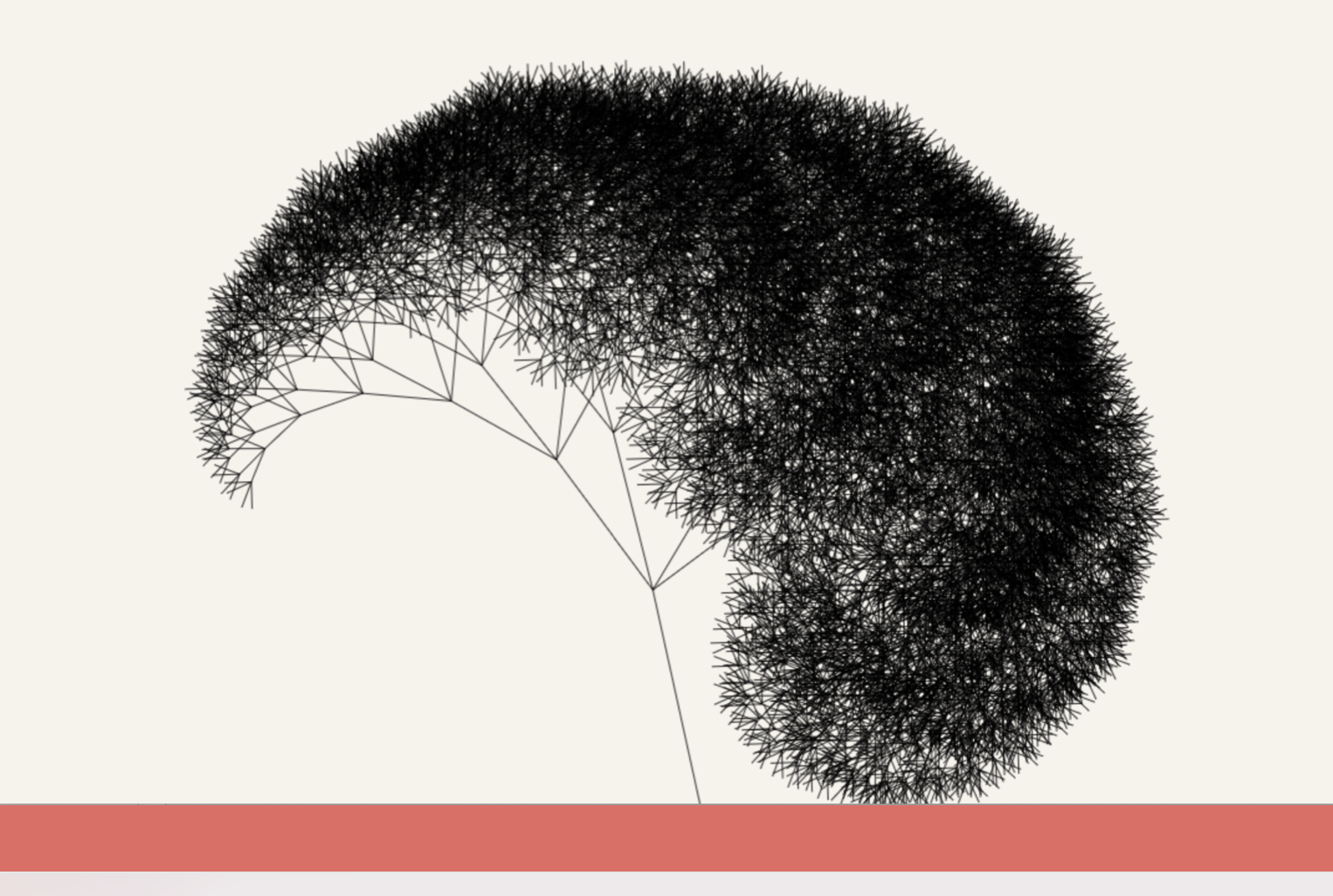but with some adaptations, definitely not performance optimized, unbalanced and decreasing branch size:

``````// draw a recursive tree
tree = (x, y, l, n=0, i=0) => {
// reduce line width on each level (wide base, thinner branches)
// increase level by one
c.lineWidth = 20 / ++i

n += Math.cos(t * .01) * .01

// calculate end coordinates based on length * sin/cos angle.
// these will be used as starting point for sub-branches
let x1 = x + l * Math.sin(n)
let y1 = y - l * Math.cos(n)

c.beginPath()
c.moveTo(x, y)
c.lineTo(x1, y1)
c.stroke()

// reduce length on each level
l *= .7

// recursivelly draw branches depending (unbalanced on purpose)
if(i<7) tree(x1, y1, l, n - 1, i)
if(i<5) tree(x1, y1, l, n + 1, i)
if(i<7) tree(x1, y1, l, n + .4, i)
}
``````

Temporarily removing the butterflies and adding calling this method on the drawing loop:

``````  c.strokeStyle = '#f8c'
c.globalAlpha = .5
tree(w/2, h/2, h/8)
``````

give us the following result: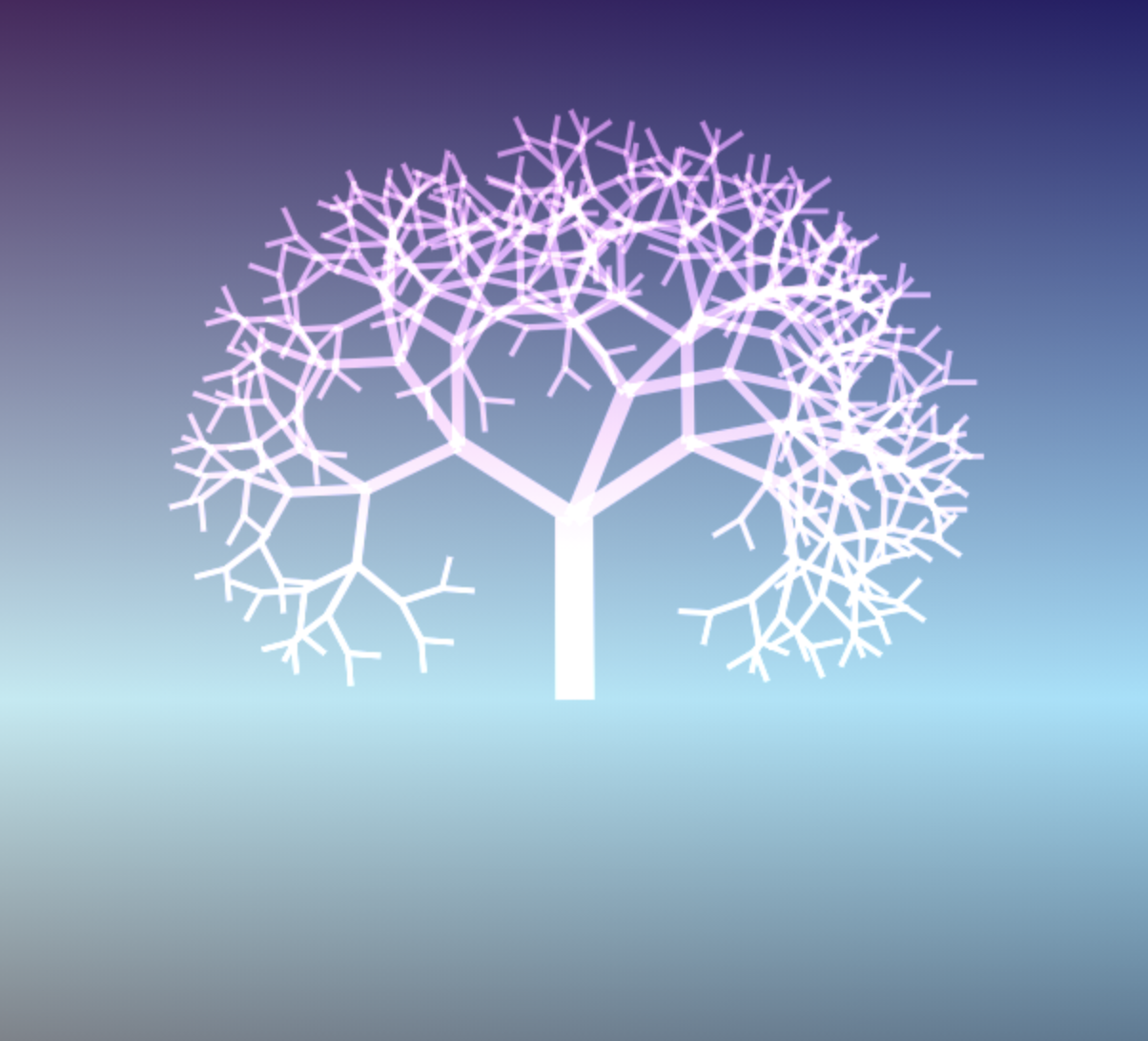Let’s now change the code to generate the butterflies at each branch node and remove the random position code from before:

``````  ...
let x1 = x + l * Math.sin(n)
let y1 = y - l * Math.cos(n)

// add butterfly coordinates (centered on the middle of the screen)
B[k++] = [x1 - w/2, y1 - h/2]

c.beginPath()
c.moveTo(x, y)
...
``````

Now it is starting to look better: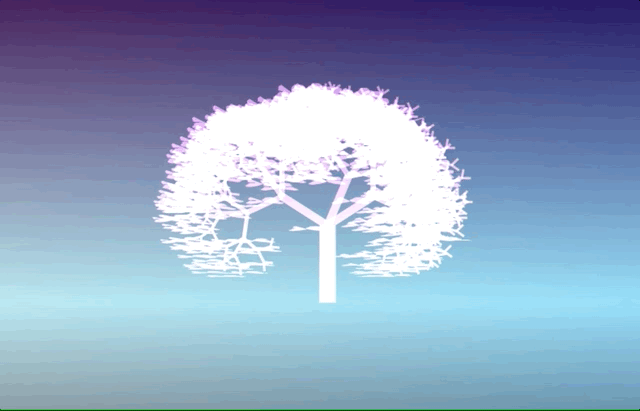with the end result, after all butterflies have departed form their original position: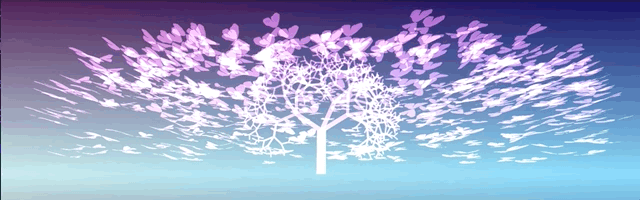Now let’s talk about the blurred reflection, I wanted to simply render everything twice with a `c.scale(1, -1)` to mirror it vertically, but it was already using too much CPU to render and I’ll miss the blur part. So decided to take an alternative approach… Use another canvas to render what has to be mirrored and draw it twice on the screen with a `blur` filter on the bottom part:

``````  // create an auxiliar canvas to draw the reflection
q = d.createElement`canvas`
v = q.getContext`2d`

// a is the original canvas
w = a.width
h = a.height

// set the auxiliar canvas to the same height as the original one
q.height = h

...

setInterval(_=>{
// clear screen and set width to both canvas
a.width = q.width = w

... render everything

// draw butterflies & tree from one canvas to the main one and their reflection
// i-- substracts from i and serves as loop condition while i != 0
for(i = 2; i--; ) {
c.save()

if (i) {
// for the refletion, set a blur filter and inverted vertical scale
c.filter = 'blur(2px)'
c.scale(1,-1)
}

c.drawImage(q,0,-i*h)
c.restore()
}

...

})
``````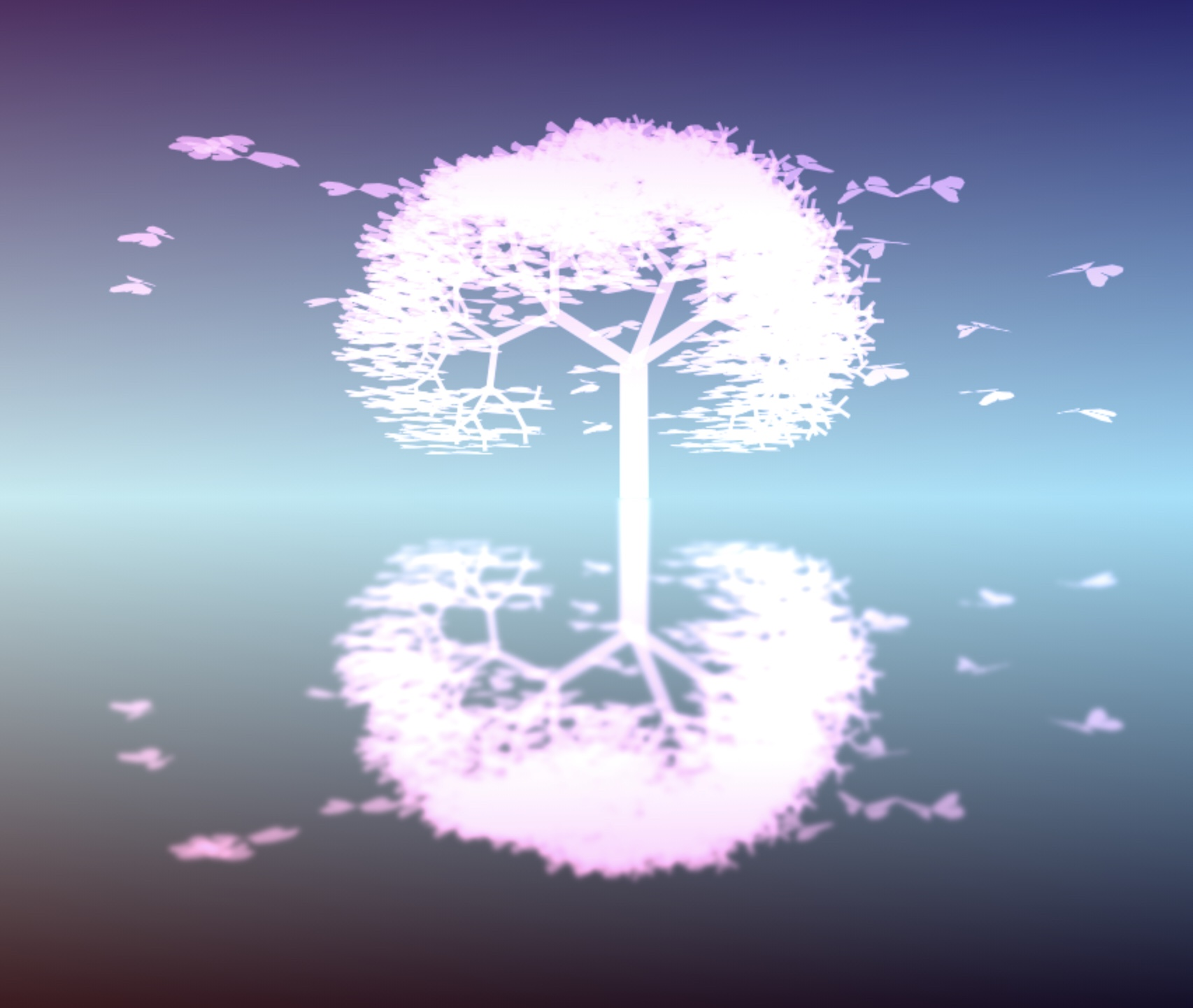One last touch I wanted to do is to randomize the flight of the butterflies, as they start flying in order right now. For this, I created a helper array with simply a randomize set of indexes:

``````//randomize indexes so butterflies start flying randomly
o = [...Array(607).keys()].sort(_ => .5 - Math.random())
``````

and update the method that draws the tree, and sets the butterfly position, to use this random index:

``````  // instead of B[k++] = [x1 - w/2, y1 - h/2]
B[o[k++]] = [x1 - w/2, y1 - h/2]
``````

Seems the random function for the sorting is not fully working on Firefox. Talking about issues with Firefox, although it is working fine on Mac, it does not seem to like any clipping, tranformation or filter together with the lighter `globalCompositeOperation`, giving a strange pinkish result on the reflection: